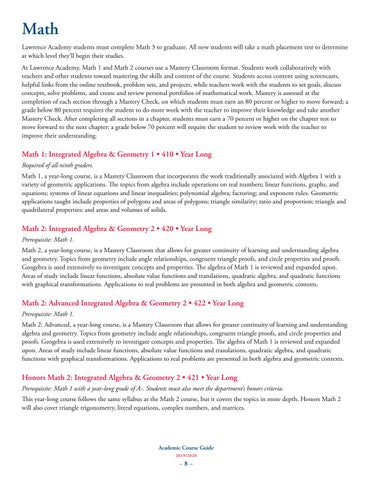Math Lawrence Academy students must complete Math 3 to graduate. All new students will take a math placement test to determine at which level they’ll begin their studies. At Lawrence Academy, Math 1 and Math 2 courses use a Mastery Classroom format. Students work collaboratively with teachers and other students toward mastering the skills and content of the course. Students access content using screencasts, helpful links from the online textbook, problem sets, and projects, while teachers work with the students to set goals, discuss concepts, solve problems, and create and review personal portfolios of mathematical work. Mastery is assessed at the completion of each section through a Mastery Check, on which students must earn an 80 percent or higher to move forward; a grade below 80 percent requires the student to do more work with the teacher to improve their knowledge and take another Mastery Check. After completing all sections in a chapter, students must earn a 70 percent or higher on the chapter test to move forward to the next chapter; a grade below 70 percent will require the student to review work with the teacher to improve their understanding.

Math 1: Integrated Algebra & Geometry 1 • 410 • Year Long Required of all ninth graders. Math 1, a year-long course, is a Mastery Classroom that incorporates the work traditionally associated with Algebra 1 with a variety of geometric applications. e topics from algebra include operations on real numbers; linear functions, graphs, and equations; systems of linear equations and linear inequalities; polynomial algebra; factoring; and exponent rules. Geometric applications taught include properties of polygons and areas of polygons; triangle similarity; ratio and proportion; triangle and quadrilateral properties; and areas and volumes of solids.

Math 2: Integrated Algebra & Geometry 2 • 420 • Year Long Prerequisite: Math 1. Math 2, a year-long course, is a Mastery Classroom that allows for greater continuity of learning and understanding algebra and geometry. Topics from geometry include angle relationships, congruent triangle proofs, and circle properties and proofs. Geogebra is used extensively to investigate concepts and properties. e algebra of Math 1 is reviewed and expanded upon. Areas of study include linear functions, absolute value functions and translations, quadratic algebra, and quadratic functions with graphical transformations. Applications to real problems are presented in both algebra and geometric contexts.

Math 2: Advanced Integrated Algebra & Geometry 2 • 422 • Year Long Prerequisite: Math 1. Math 2: Advanced, a year-long course, is a Mastery Classroom that allows for greater continuity of learning and understanding algebra and geometry. Topics from geometry include angle relationships, congruent triangle proofs, and circle properties and proofs. Geogebra is used extensively to investigate concepts and properties. e algebra of Math 1 is reviewed and expanded upon. Areas of study include linear functions, absolute value functions and translations, quadratic algebra, and quadratic functions with graphical transformations. Applications to real problems are presented in both algebra and geometric contexts.

Honors Math 2: Integrated Algebra & Geometry 2 • 421 • Year Long Prerequisite: Math 1 with a year-long grade of A-. Students must also meet the department’s honors criteria. is year-long course follows the same syllabus as the Math 2 course, but it covers the topics in more depth. Honors Math 2 will also cover triangle trigonometry, literal equations, complex numbers, and matrices.